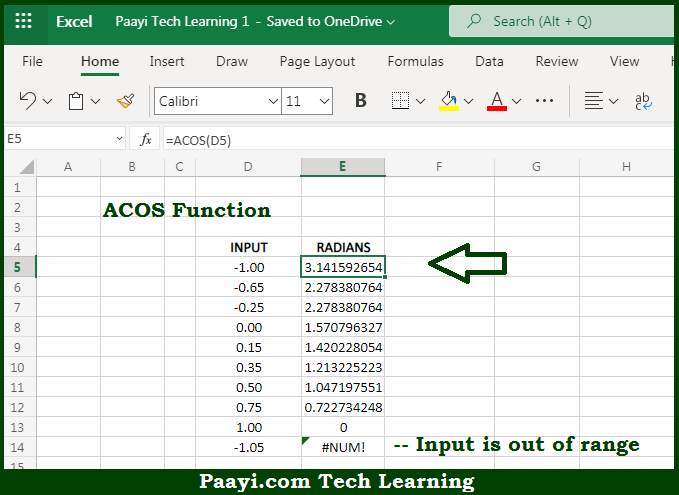# Learn How to Use Microsoft Excel ACOS Function

Written by | 0 Comments | 447 Views

In this article, you will learn how to use the Microsoft Excel ACOS function and its prime function in Microsoft Excel. You will also get to know the Microsoft Excel ACOS function return value and syntax with the help of some examples.

Microsoft Excel ACOS Function

The main function of the Microsoft Excel ACOS function is to get inverse cosine values of a value, in radians. That implies, with the help of the ACOS function you can able to return the inverse cosine of the number. It should be noted that the ACOS function is the inverse of the COS function and you need to input the values in the range of -1 to 1. So, with the help of the ACOS function, you can able to get inverse cosine values of a value, in radians.

Return Value of ACOS Function

The return value will be the angle in radians.

Syntax of ACOS Function

=ACOS(number)

Where the arguments:

• number: This is the number or value of which you want to get the inverse cosine, and it should be between the range of -1 and 1.

## How to Use Microsoft Excel ACOS Function?So we know that Microsoft Excel ACOS function you can able to get inverse cosine values of a value, in radians. That implies, with the help of the ACOS function you can able to return the inverse cosine of the number. It should be noted that the ACOS function is the inverse of the COS function and you need to input the values in the range of -1 to 1. So, with the help of the ACOS function, you can able to get inverse cosine values of a value, in radians.